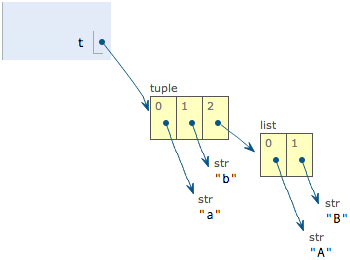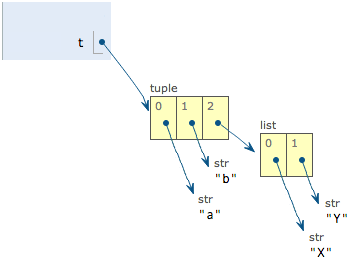# Python的tuple和list

## list

list是一种有序的集合，可以随时添加和删除其中的元素。

``````a = [1, 2, 3, 4, 5, 6, 7, 8, 9, 10]
b = [2, 5, 8, 11, 0]

# intersection
intersection = list(set(a).intersection(set(b)))
# union
union = list(set(a).union(set(b)))
# difference
difference = list(set(a).difference(set(b)))
``````

append、extend和insert:
– append() 追加单个元素到List的尾部，只接受一个参数，参数可以是任何数据类型，被追加的元素在List中保持着原结构类型

``````In : l1 = [1,2,3,4]
In : l2 = [5,6,7]
In : l1.append(l2)
In : l1
Out: [1, 2, 3, 4, [5, 6, 7]]
``````
• extend() 将一个列表中每个元素分别添加到另一个列表中，只接受一个参数
``````In : l3 = [1,2,3,4]
In : l4 = [5,6,7]
In : l3.extend(l4)
In : l3
Out: [1, 2, 3, 4, 5, 6, 7]
``````
• insert是在某个位置插入一个元素
``````In : l5 = [1,2,3,4]
In : l5.insert(1, 9)
In : l5
Out: [1, 9, 2, 3, 4]
``````

1、对于 不可 变类型 Number String Tuple，浅复制仅仅是地址指向，不会开辟新空间。
2、对于 可 变类型 List、Dictionary、Set，浅复制会开辟新的空间地址(仅仅是最顶层开辟了新的空间，里层的元素地址还是一样的)，进行浅拷贝
3、浅拷贝后，改变原始对象中为可变类型的元素的值，会同时影响拷贝对象的；改变原始对象中为不可变类型的元素的值，只有原始类型受影响。 （操作拷贝对象对原始对象的也是同理）

``````import copy

l1 = [11, 12]
l2 = [21, 22]
num = 555

allOne = [l1, l2,num]

# 浅拷贝，创建出一个对象，并把旧对象元素的 引用地址 拷贝到新对象当中。
# 也就是说，两个对象里面的元素通过浅拷贝指向的还是同一个地址
allOne2 = copy.copy(allOne)

l1 = 16   # 此处修改，会使得 allOne 和 allOne2的第0个元素的值都发生改变，因为l1是List，是可变对象
allOne = 666   # 此处修改，只会allOne的num的值，因为不可变对象一旦重新复制，地址就会发生改变。（不可变嘛）

num = 777   # 此处不会改变 allOne 和 allOne2的值，因为相当于 777 复制给一个全新的地址，这个num跟其他num已经没关系了

print(allOne)
print(allOne2)

print("id allOne:"+str(id(allOne)))
print("id allOne:"+str(id(allOne)))
print("id allOne:"+str(id(allOne)))
print("id allOne:"+str(id(allOne)))

print("===")
print("id allOne2:"+str(id(allOne2)))
print("id allOne2:"+str(id(allOne2)))
print("id allOne2:"+str(id(allOne2)))
print("id allOne2:"+str(id(allOne2)))
``````

``````[[16, 12], [21, 22], 666]
[[16, 12], [21, 22], 555]
id allOne:4467341640
id allOne:4471819912
id allOne:4467342920
id allOne:4466847696
===
id allOne2:4471820232
id allOne2:4471819912
id allOne2:4467342920
id allOne2:4466081744
``````

1、浅拷贝，除了顶层拷贝，还对子元素也进行了拷贝（本质上递归浅拷贝）
2、经过深拷贝后，原始对象和拷贝对象所有的子元素地址都是独立的了
3、可以用分片表达式进行深拷贝
4、字典的copy方法可以拷贝一个字典

``````import copy

l1 = [11, 12]
l2 = [21, 22]
num = 555

allOne = [l1, l2,num]

# 浅拷贝，除了顶层拷贝，还对子元素也进行了拷贝（本质上递归浅拷贝）
# 经过深拷贝后，原始对象和拷贝对象所有的元素地址都没有相同的了
allOne2 = copy.deepcopy(allOne) # copy.deepcopy 深拷贝

allOne = [113,114]
allOne2 = [227,228]

print(allOne)
print(allOne2)

print("id allOne:"+str(id(allOne)))
print("id allOne:"+str(id(allOne)))
print("id allOne:"+str(id(allOne)))
print("id allOne:"+str(id(allOne)))

print("===")
print("id allOne2:"+str(id(allOne2)))
print("id allOne2:"+str(id(allOne2)))
print("id allOne2:"+str(id(allOne2)))
print("id allOne2:"+str(id(allOne2)))
``````

``````[[11, 12], [113, 114], 555]
[[11, 12], [21, 22], [227, 228]]
id allOne:4549589640
id allOne:4554067720
id allOne:4554067848
id allOne:4548329424
===
id allOne2:4554067912
id allOne2:4554067784
id allOne2:4554067592
id allOne2:4554100808
``````

## tuple

``````>>> t = (1)
>>> t
1

>>> t = (1,)
>>> t
(1,)
``````

``````>>> t = ('a', 'b', ['A', 'B'])
>>> t = 'X'
>>> t = 'Y'
>>> t
('a', 'b', ['X', 'Y'])
````````````>>> s = ('Jack', 21, 'male', '5788666@qq.com')
``````

``````>>> from collections import namedtuple
>>> Student = namedtuple('Student', ['name', 'age', 'sex', 'email'])
>>> s = Student('Jim', 21, 'male', '123@qq.com')
>>> s.name
'Jim'
>>> s.age
21
``````